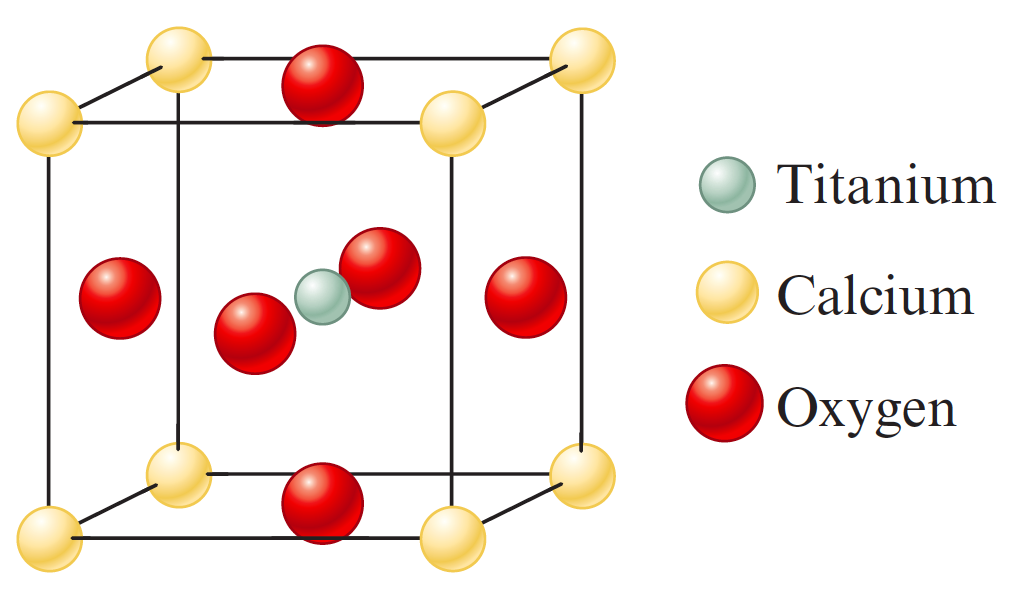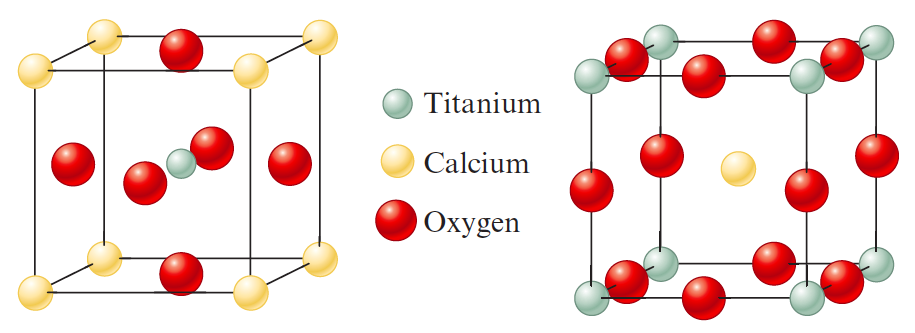# Problem: Perovskite is a mineral containing calcium, titanium, and oxygen. Two different representations of the unit cell are shown below. Show that both these representations give the same formula and the same number of oxygen atoms around each titanium atom.

###### FREE Expert Solution

Since perovskite follows a cubic unit cell, we can determine its molecular formula based on the number of atoms present in the unit cell.

For the first structure:94% (147 ratings)###### Problem Details

Perovskite is a mineral containing calcium, titanium, and oxygen. Two different representations of the unit cell are shown below. Show that both these representations give the same formula and the same number of oxygen atoms around each titanium atom.